# Precalculus : Geometric Vectors

## Example Questions

1 3 Next →

### Example Question #171 : Matrices And Vectors

Subtract: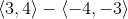Possible Answers: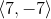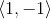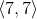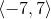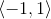Correct answer:Explanation:

Subtract the first value of the first vector, and the second value of the first vector with the second value of the second vector.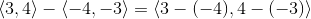Double negative signs are converted to a positive sign.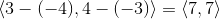### Example Question #172 : Matrices And Vectors

Simplify: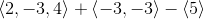Possible Answers: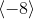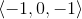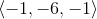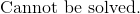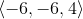Correct answer:Explanation:

The dimensions of the vectors are not the same.  Placeholders cannot be added to a vector.  Therefore, the values of the vectors cannot be added.

The correct answer is:### Example Question #21 : Evaluate Geometric Vectors

Find the norm of the vector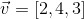.

Possible Answers: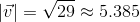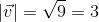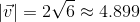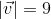Correct answer:Explanation:

We find the norm of a vector by finding the sum of each element squared and then taking the square root.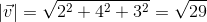.

### Example Question #22 : Evaluate Geometric Vectors

Find the norm of the vector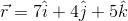.

Possible Answers: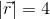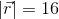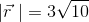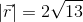Correct answer:Explanation:

We find the norm of a vector by finding the sum of each component squared and then taking the square root of that sum.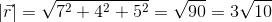### Example Question #23 : Evaluate Geometric Vectors

Find the norm of the vector: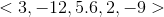Possible Answers: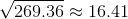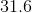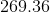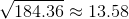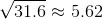Correct answer:Explanation:

The norm of a vector is also known as the length of the vector. The norm is given by the formula: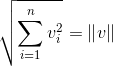.

Here, we have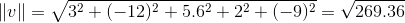,

the correct answer.

### Example Question #24 : Evaluate Geometric Vectors

Find the norm of vector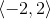.

Possible Answers: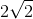Correct answer:Explanation:

Write the formula to find the norm, or the length the vector.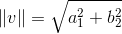Substitute the known values of the vector and solve.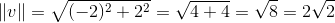### Example Question #25 : Evaluate Geometric Vectors

Find the norm (magnitude) of the following vector: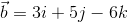Possible Answers: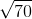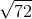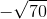Correct answer:Explanation:

Use the following equation to find the magnitude of a vector: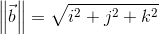In this case we have:So plug in our values: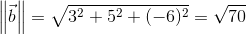So: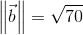### Example Question #26 : Evaluate Geometric Vectors

Find the product of the vector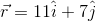and the scalar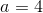.

Possible Answers: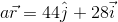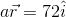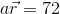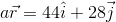Correct answer:Explanation:

When multiplying a vector by a scalar we multiply each component of the vector by the scalar and the result is a vector: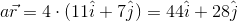1 3 Next →

### All Precalculus Resources